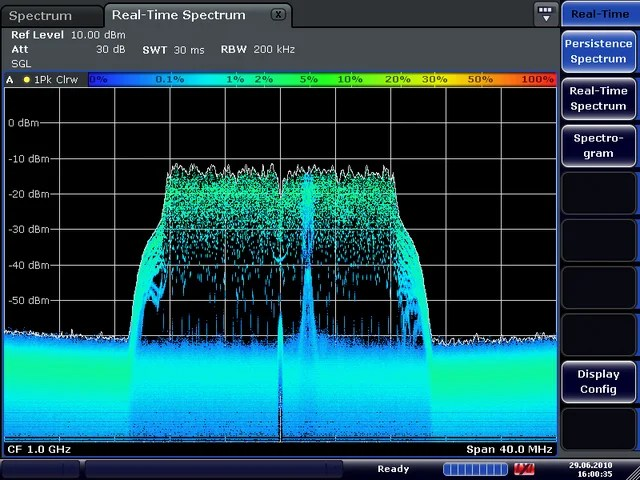# What is THD and How to calculate THDTHD or Total Harmonic Distortion (THD) is a value that indicates how the system has how much harmonic. When THD = 100% or 1.0 mean the system has 100% harmonic and THD = 0 mean No harmonic in the system.

In sound application, less THD means equipment has accurate, less noise and sound quality is very good.

In Power application, less THD means the system has a minimum loss, low heat loss. This is good for the system.

We can define THD of Voltage and Current by the formula. THD has 2 types of standard.

1) THD definition from IEC standard
Total harmonic distortion, THD (abbreviation) :
The ratio of the Root mean square (RMS) value of the harmonic content of an alternating quantity to the RMS value of the fundamental component of the quantityWhen

Vh is Voltage RMS Harmonic order, Order 2 to Order n

V1 is Voltage RMS fundamental or V order 1 (no Harmonics)

h is an order of Harmonics Order 2 to Order n (h≥2)When

Ih is Current RMS Harmonic order, Order 2 to Order n

I1 is Current RMS fundamental or I order 1 (no Harmonics)

h is an order of Harmonics Order 2 to Order n (h≥2)

The THD formula above, which is defined from IEC standard, it has the opportunity for value of THD more than 100% (Harmonicas value are combined more than fundamental order).

2) THD calculationwhen

V is Voltage RMS include Harmonics and Fundamental order

I is Current RMS include Harmonics and Fundamental order

The THD formula, it has no opportunity for value of THD more than 100%. Because the num (include harmonics) has more value than den value.

From THD definition THD is ratio but most people are using THD value as percent and use formula follow IEC standard.

Example of THD calculation (IEC standard)

If Power system has Current Harmonic

Fundamental current = 100 A

5th Harmonic component  =  20 A

7th Harmonic component = 12 ACurrent THD  = 0.23 or 23%.